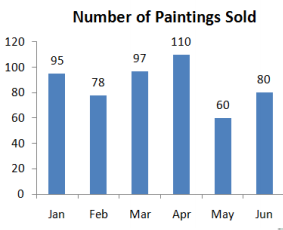• Save

# SSC CGL Maths Important Questions 2021

Vikram SinghLast week 161 Views
NEWQ :

A man borrows some money at 3% simple interest per annum and lends it to somebody at 5% interest to be compounded annually. By this he makes a profit of Rs.541 at the end of 3 years. The money he borrowed was-

(A) Rs. 15800

(B) Rs. 18400

(C) Rs. 8000

(D) Rs. 12000

Q :

Present age of Ram and Shyam are in the ratio of 4: 5 respectively. Five year hence the ratio of their age becomes 5:6 respectively. What is Ram’s present age?

(A) 25 years

(B) 22 years

(C) 20 years

(D) 30 years

Q :

Water flows in to a tank 15 m.×10 m. through a rectangular pipe 2 m.×1.5 m. at 20 km./hr. In how much time will the water level in the tank rise by 3m.?

(A) 23 seconds

(B) 27 seconds

(C) 15 seconds

(D) 19 seconds

Q :

Solve the following equation 24 + 4 ÷ 4

(A) 28

(B) 6

(C) 25

(D) 7

Q :

A boat running upstream takes 7 hours and 40 minutes to cover a certain distance while it takes 5 hours to cover the same distance downstream. What is the ratio between the speed of the current and speed of the boat respectively?

(A) 13: 4

(B) 17: 4

(C) 19: 4

(D) 4: 19

Q :

The sum of two numbers is 232 and their HCF is 29. What is the numbers of such pairs of numbers satisfied the above condition?

(A) 0

(B) 2

(C) 3

(D) 1

Q :

The slant height of a right circular cone is 15 m and its height is 9 m. The area of its curved surface, is:

(A) 169 π m2

(B) 172 π m2

(C) 165 π m2

(D) 180 π m2

Q :

The bar graph shows the number of painting sold an art gallery in each month from January to June.How many paintings does it need to sell in July for the 7 month average to be 90?

(A) 115

(B) 85

(C) 90

(D) 110

Q :

The difference between a fraction and its reciprocal is 9/11. If the cubes of both the fraction and its reciprocal are considered, what will be the difference between them?

(A) 3996/1331

(B) -1331/2538

(C) 729/1331

(D) -2538/1331

Q :

The average weight of 8 people increase by 2.5 kg when a new person comes in place of one of them weighting 75 kg. What is the weight of the new person?

(A) 95 kg

(B) 75 kg

(C) 85 kg

(D) 65 kg